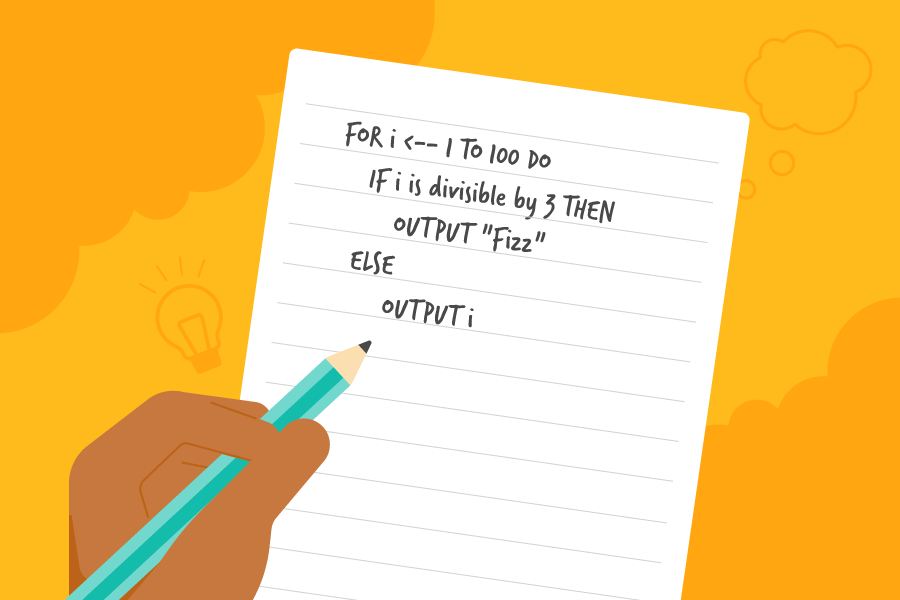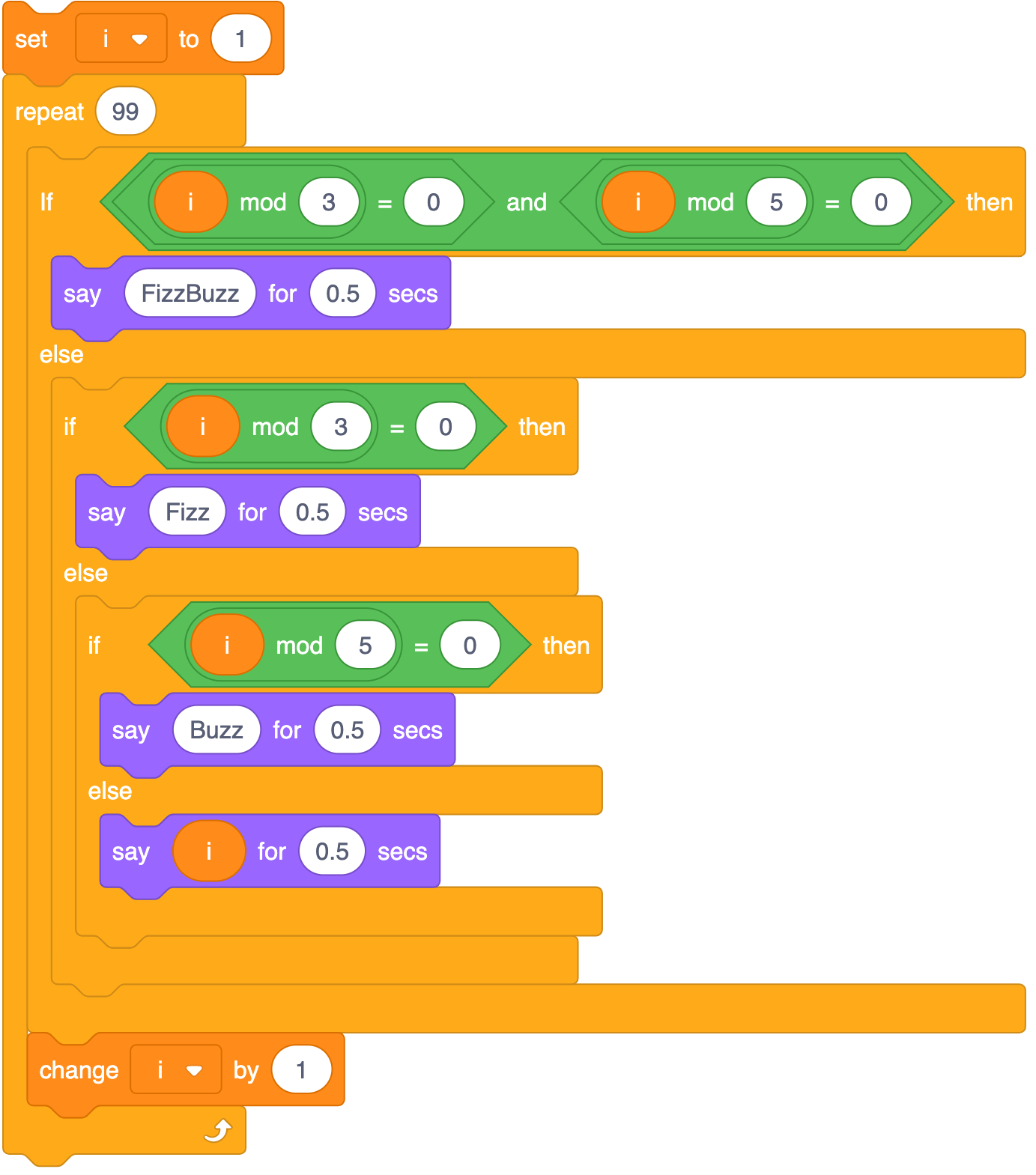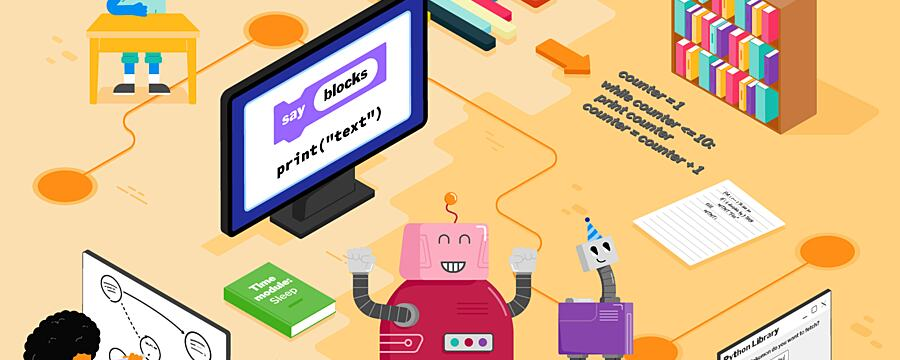﻿ Introducing pseudocode
•# Introducing pseudocode

How to use pseudocode to plan your programsWhen you discuss how a program works, showing the code may not be the easiest way of explaining it — especially if the person you are talking to is less familiar with the programming language you are using. In these situations you may want to express your program in pseudocode.
Pseudocode is a way of expressing an algorithm without conforming to specific syntax rules. By learning to read and write pseudocode, you can easily communicate ideas and concepts to other programmers, even though they may be using completely different languages. What’s more, algorithmic solutions to many problems are often provided in pseudocode on sites such as Wikipedia, meaning an ability to translate between pseudocode and a given programming language is a valuable skill.
There is no such thing as correct pseudocode, although there are a few generally accepted notations that are widely understood by programmers. For example x <-- 10 to assign a new value of 10 to the variable x, having first created the variable x if it didn’t already exist.
In England, GCSE exam boards have different approaches to using pseudocode, so it is worth checking your exam board’s specification before you start to teach it. To overcome this, the Teach Computing team have created a version of pseudocode to use with the Teach Computing Curriculum lesson resources which cover all the GCSE specifications. You can download this resource using the pdf document attached to this step.
Now you are going to have a look at a simple pseudocode example and see the equivalent code in both Scratch and Python. You could adapt this to help your students learn to translate between pseudocode, Scratch, and Python.

## FizzBuzz

FizzBuzz is a common programming challenge with hundreds of different solutions in many languages. The challenge is as follows:
For every number from one to one hundred, output Fizz if the number is divisible by three, output Buzz if the number is divisible by five, and output FizzBuzz if the number is divisible by both three and five. If none of these conditions match, then just output the number.
You are now going to look at one possible pseudocode interpretation of this challenge:
FOR i <-- 1 TO 100 DO IF i is divisible by 3 AND i is divisible by 5 THEN OUTPUT "FizzBuzz" ELSE IF i is divisible by 3 THEN OUTPUT "Fizz" ELSE IF i is divisible by 5 THEN OUTPUT "Buzz" ELSE OUTPUT i
Here, you start by using a loop to iterate over all the numbers. Then IF, ELSE IF, and ELSE control statements are used to decide the output depending on the number.
This code can easily be translated into any programming language.
Here’s the same algorithm in Scratch:You might already begin to see how a program to address this type of problem can become overly complicated in a language like Scratch. You can discuss with your students where it is necessary to nest blocks within blocks to make the program work.
Here’s an example of a FizzBuzz algorithm in Python:
for i in range(1,100): if i % 3 == 0 and i % 5 == 0: print('FizzBuzz') elif i % 3 == 0: print('Fizz') elif i % 5 == 0: print('Buzz') else: print(i)
You’ll probably notice that this is much closer to the pseudocode. It is only one of many possible solutions to the problem, and in a text-based language your superpowers can be greatly expanded once you know a few tips and tricks.
For instance, this program could be written in a single line:
print("\n".join(["Fizz" * (i % 3 == 0) + "Buzz" *(i % 5 == 0) or str(i) for i in range(1, 100)]))
You don’t have to understand this code, and it is not how you’d present this program to beginners, but it provides an example of how more concise you can be with a text-based language compared to block-based language.

## Try it out

Now it’s your turn to have a go at converting some pseudocode.
A prime number is one that is only divisible by the number one and itself. You can test if a number is prime by trying to divide it by every number from two onward.
Try translating this pseudocode into Python (you can translate it to Scratch first if you like):
number <-- 17prime <-- TRUEFOR i <-- 2 TO number IF number is divisible by i THEN prime <-- FALSEIF prime = TRUE: OUTPUT "prime"ELSE OUTPUT "not prime"
Check that your algorithm works by changing the value of number to various prime and non-prime numbers, and running your program.
How did you get on? What are your thoughts on the effectiveness of pseudocode in supporting those new to programming?

#### Scratch to Python: Moving from Block- to Text-based Programming